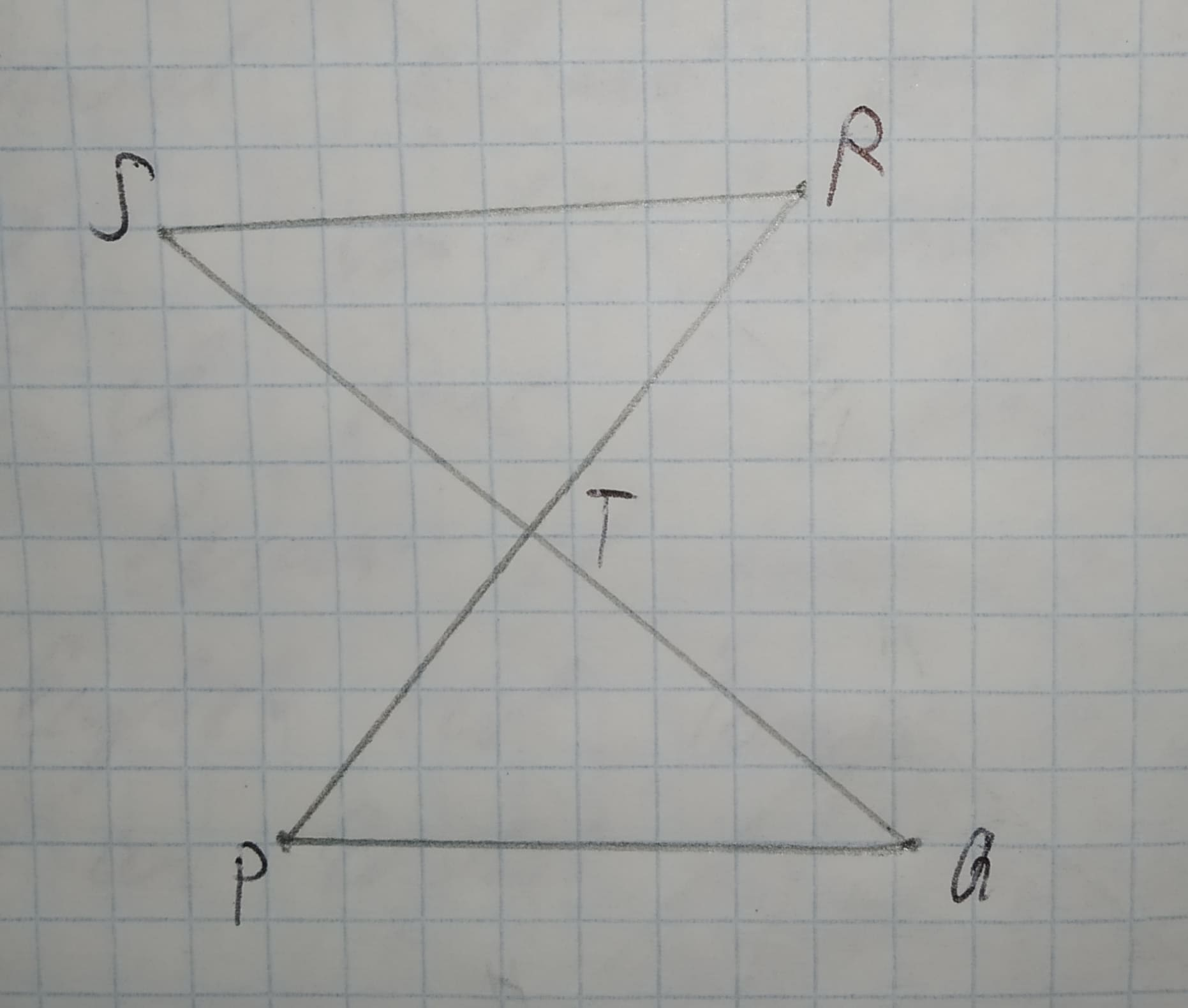# Find the triangle congruence (SSS SAS AAS HL)12210203761.jpgGiven: bar(SQ) and bar(PR) bisect each other.Ramsey 2021-02-25 Answered

Find the triangle congruence (SSS SAS AAS HL)Given: bar(SQ) and bar(PR) bisect each other.

You can still ask an expert for help

## Want to know more about Congruence?

• Questions are typically answered in as fast as 30 minutes

Solve your problem for the price of one coffee

• Math expert for every subject
• Pay only if we can solve itDelorenzoz
Step 1
In given triangle $\mathrm{△}STR\phantom{\rule{1em}{0ex}}\text{and}\phantom{\rule{1em}{0ex}}\mathrm{△}PTQ$ we have
SQ and PR are bisect each other then we have
Step 2
ST = TQ ........(1)
RT = TP ........(2)
$\mathrm{\angle }STR=\mathrm{\angle }PTQ$ ........(3)
( Vertical opposite angle)
Then from 1 , 2 and 3 , we have
$\mathrm{△}STR$ congruence $\mathrm{△}PTQ$ by SAS rule.